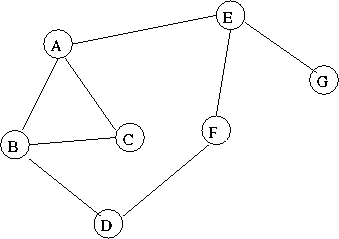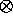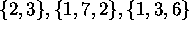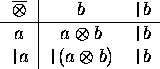# Special Cases of Parallel Prefix Networks

Tom Kelliher, CS 315

Mar. 29, 1999

### From Last Time

Parallel prefix on a 2-D array.

### Outline

1. Parallel prefix on arbitrary networks.

2. Parallel segmented prefix computations.

### Coming Up

Carry save, multiplication, convolution.

# Parallel Prefix on General Graphs

Consider an arbitrary graph/network:Form the breadth-first spanning tree from vertex A.

Properties of the breadth-first spanning tree:

1. Finds shortest path from distinguished vertex to all other vertices.

2. Depth is at most diameter of graph. (Find an example where the depth is less than the diameter of the graph.)

3. Not necessarily binary.

Observation: parallel prefix algorithm can be implemented with slowdown at most the diameter of the graph.

Idea: each vertex of the network simulates a leaf and an internal vertex of the tree.

1. Draw for example graph.

2. Do an example.

# Segmented Prefix Computation

Definition:

A sequence of prefix computations that use the same associative operator, but on disjoint sets of data.

Example: Addition on.

1. Obviously, we can solve using three prefix computations. With a trick, we can do it in one.

2. The trick: use `|` as a barrier symbol at the start of each set of data.

3. The data set: `|`2, 3, `|`1, 7, 2, `|`1, 3, 6.

Redefine our operator:``Forget about everything to the left of the barrier.''

4. Run the example.

5. What is the running time of the parallel segmented prefix computation?

Thomas P. Kelliher
Mon Mar 29 10:41:52 EST 1999
Tom Kelliher